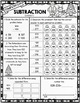# Third Grade Winter Math Packet Winter WorksheetsResource Type
File Type

PDF

(26 MB|74 pages)
Product Rating
4.0
(4 Ratings)
Standards
• Product Description
• StandardsNEW

Looking for the perfect math packet for your students to complete over Winter Break? This 3rd Grade Winter Math Packet is the perfect product to keep your students practicing the third grade skills over the holiday. This Winter Break Math Packet has everything you need with no prep involved! Just print and go! These worksheet pages are designed with various winter themes ( Christmas, Hanukkah, Winter, Snowmen, Elves & more) to keep your students interested in the packet while reviewing the third grade content. Th Product includes 30 pages of practice

This winter math packet can be used during those last few weeks before winter break or can be the perfect option to send home over the holidays!

This product contains resources that review 3rd Grade VA SOL's and Common Core Standards. There are no page numbers listed so you can pick and choose what pages you want to include in your students packets.

Answer Key is included to make this packet easy for both parents and teachers!

Check out the preview to view the full product!

VA Math SOL's: 3.1a, 3.1b, 3.1c, 3.3a, 3.3b, 3.4a, 3.4b, 3.4c, 3.4d, 3.9a, 3.9b, 3.9c, 3.11, 3.12a, 3.12b, 3.12c, 3.13

Winter Math Packet Content:

Place Value

• Number Form
• Rounding Numbers
• Values & Digits
• Comparing Numbers

Computation

• 3 Digit Subtraction
• 4 Digit Subtraction
• Addition & Subtraction Word Problems
• Multiplication Facts
• Multiplication Strategies
• Division Facts
• Division Strategies
• Multiplication & Division Word Problems
• Multi Step Word Problems

Time

• Time to the Hour
• Time to the Minute
• Elapsed Time to the Hour
• Elapsed Time to the Minute
• Time Equivalents
• Calendar

Geometry

• Subdividing Polygons
• Partitioning Shapes
• Lines & Angles
• Congruent Shapes
Partition shapes into parts with equal areas. Express the area of each part as a unit fraction of the whole. For example, partition a shape into 4 parts with equal area, and describe the area of each part as 1/4 of the area of the shape.
Understand that shapes in different categories (e.g., rhombuses, rectangles, and others) may share attributes (e.g., having four sides), and that the shared attributes can define a larger category (e.g., quadrilaterals). Recognize rhombuses, rectangles, and squares as examples of quadrilaterals, and draw examples of quadrilaterals that do not belong to any of these subcategories.
Solve two-step word problems using the four operations. Represent these problems using equations with a letter standing for the unknown quantity. Assess the reasonableness of answers using mental computation and estimation strategies including rounding.
Fluently multiply and divide within 100, using strategies such as the relationship between multiplication and division (e.g., knowing that 8 × 5 = 40, one knows 40 ÷ 5 = 8) or properties of operations. By the end of Grade 3, know from memory all products of two one-digit numbers.
Determine the unknown whole number in a multiplication or division equation relating three whole numbers. For example, determine the unknown number that makes the equation true in each of the equations 8 × ? = 48, 5 = __ ÷ 3, 6 × 6 = ?.
Total Pages
74 pages
Included
Teaching Duration
N/A
Report this Resource to TpT
Reported resources will be reviewed by our team. Report this resource to let us know if this resource violates TpT’s content guidelines.